# NCERT Solutions For Class 6 Maths Symmetry Exercise 13.3

NCERT Solutions For Class 6 Maths Symmetry Exercise 13.3

### NCERT Solutions For Class 6 Maths Symmetry Exercise 13.3

NCERT Solutions For Class 6 Maths Chapter 13 Symmetry Ex 13.3

Exercise 13.3

Ex 13.3 Class 6 Maths Question 1.
Find the number of lines of symmetry in each of the following shapes. How will you check your answer?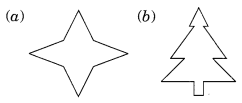Solution:
(a) The given figure has 4 lines of symmetry.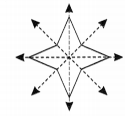(b) The given figure has only one line of symmetry.(c) The given figure has two line of symmetry.(d) The given figure has two lines of symmetry.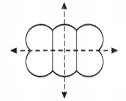(e) This figure has only one line of symmetry.(f) The given figure has two lines of symmetry.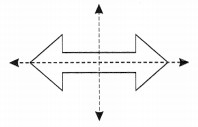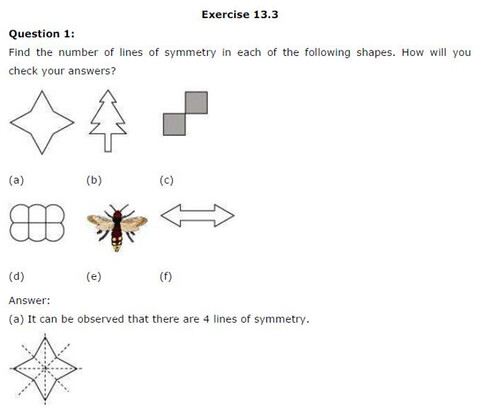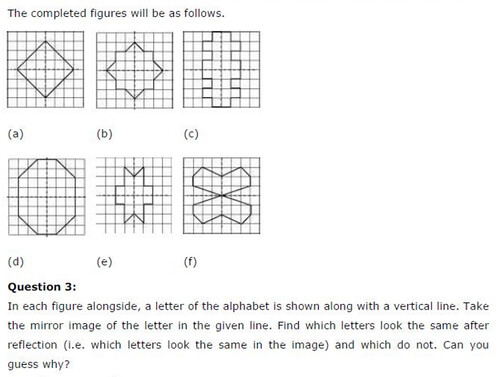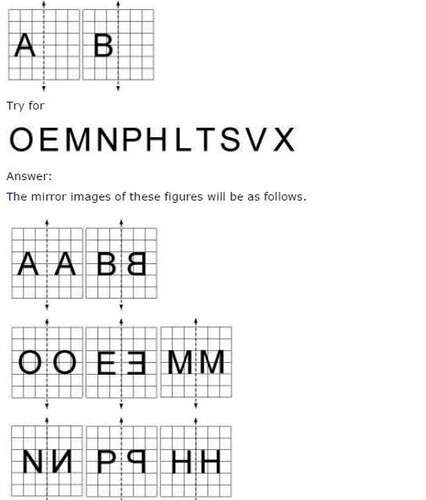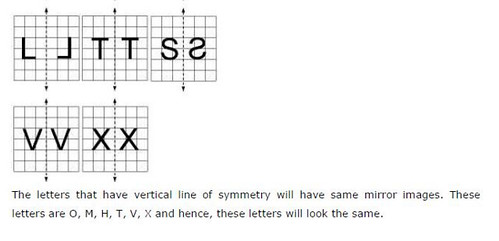## SabDekho

The Complete Educational Website